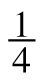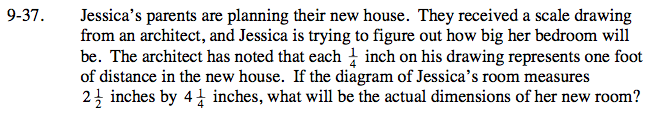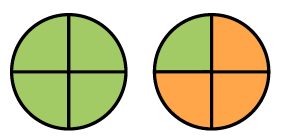Home > CC2 > Chapter 9 > Lesson 9.1.2 > Problem9-37

9-37.

. Jessica’s parents are planning their new house. They received a scale drawing from an architect, and Jessica is trying to figure out how big her bedroom will be. The architect has noted that eachinch on his drawing represents one foot of distance in the new house. If the diagram of Jessica’s room measures 2inches by 4inches, what will be the actual dimensions of her new room? Homework Help ✎$\text{How many }\frac{1}{4}\text{ inches are in the }2\frac{1}{2}$

$\text{ and }4\frac{1}{4}\text{ inch measurements?}\;\;\;\;\;\;\;\;$

For example, say Jessica's bathroom is represented as a rectangle measuring 1 and one quarter inches by 2 inches. Using the same scale, you can find the actual dimensions of her new bathroom.

The diagram below visually shows that there are 5 one fourths in one and one fourth inches. Now draw the same thing for two inches.There are 5 one fourths in one and one fourth and 8 one fourths in two.

Now you can use the distance conversion and multiplication to find the actual dimensions of the bathroom. Since one-fourth inch is equivalent to 1 foot, Jessica's bathroom will be 5 feet by 8 feet.

Can you use a similar strategy to find the dimensions of Jessica's bedroom? Drawing a diagram may help!

Jessica's bedroom will be 10 feet by 17 feet.